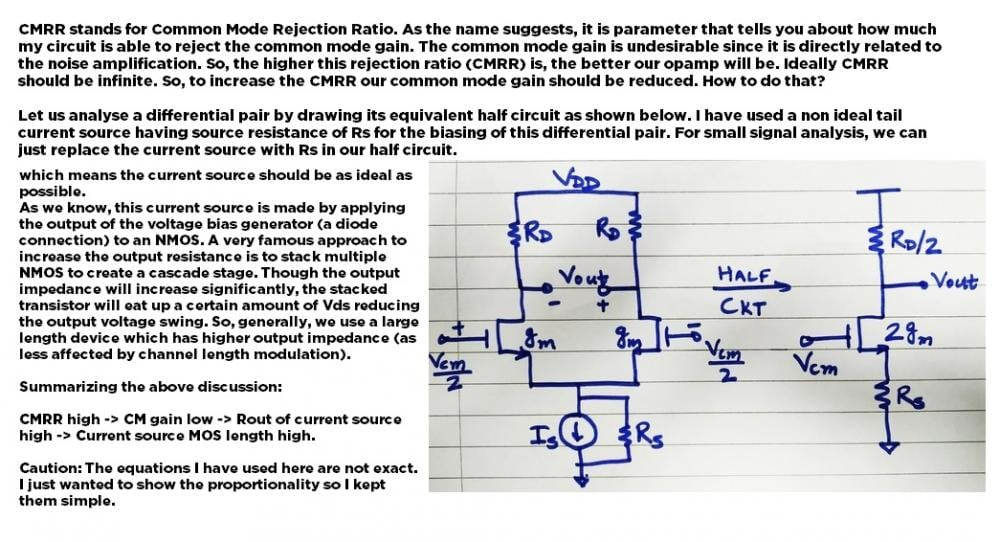# What is CMRR in Opamp

4 posts / 0 newOffline

Joined: Aug 16, 2016

Replies: 997
What is CMRR in Opamp
June 19, 2018 - 5:24pm

Why is this CMRR considered to be important, what should I check about this while selecting a Op-Amp?Offline

Joined: May 19, 2015

Replies: 213

First let me give you the standard definition..

CMRR Stands for Common Mode Rejection Ratio, which can be defined as "The ration between the Differential Mode voltage gain (Ad) and the Common Mode voltage gain (Ac)"

Now, to understand things we should know what is this Ad,Ac and why is its ratio important in selecting an Op-Amp.

As we know op-Amp has two input pins, the difference in the voltage between these two pins is called Differential mode Voltage Gain (Ad) and the common voltage between these two pins is called Common Mode Voltage gain (Ac).

The output of an Op-Amp will always be the sum of Differential mode Voltage Gain (Ad) and the Common Mode Voltage gain (Ac).

So, for any Op-Amp it is always advantages to have high value of Ad and low value of Ac. A term that relates to these two voltage gains is CMRR.

Better an Op-Amp is the higher the value of Ad will be and thus the value of CMRR will also be high. The CMRR value will be so big and hence it is always measured in dB and its value should be high for good Op-Amps

For example if CMRR if CMMR is 10000, then CMMR in db = 20log(10000)=80dbOffline

Joined: Nov 07, 2019

Replies: 124

The op amp common-mode rejection ratio (CMRR) is the ratio of the common-mode gain to differential-mode gain. For example, if a differential input change of Y volts produces a change of 1 V at the output, and a common-mode change of X volts produces a similar change of 1 V, then the CMRR is X/Y.

If a signal is applied equally to both inputs of an op amp, so that the differential input voltage isunaffected, the output should not be affected. In practice, changes in common mode voltage will produce changes in output. The op amp common-mode rejection ratio (CMRR) is the ratio of the common-mode gain to differential-mode gain. For example, if a differential input change of Y volts produces a change of 1 V at the output, and a common-mode change of X volts produces a similar change of 1 V, then the CMRR is X/Y. When the common-mode rejection ratio is expressed in dB, it is generally referred to as common-mode rejection (CMR)—please note that there is very little consistency in this throughout the semiconductor industry with regards to the use of dB or ratio values for CMR or CMRR.Offline

Joined: Jul 16, 2020

Replies: 2

Just check this image, Thank you